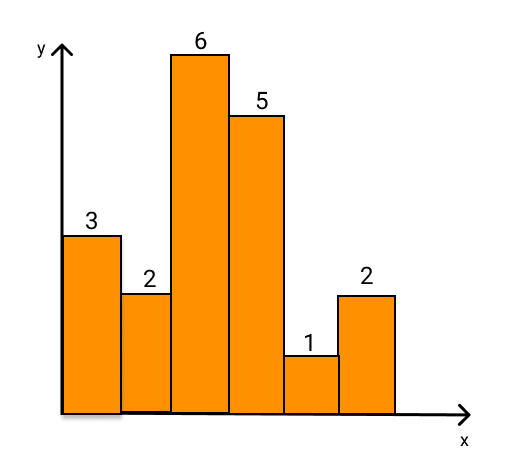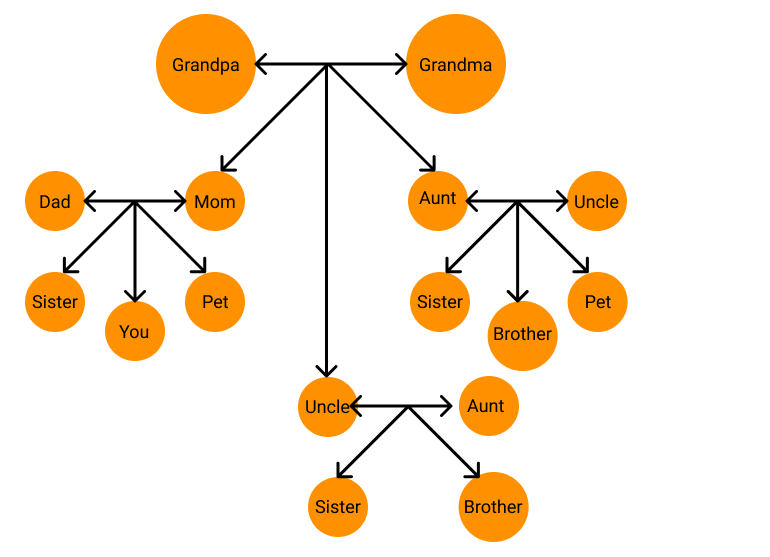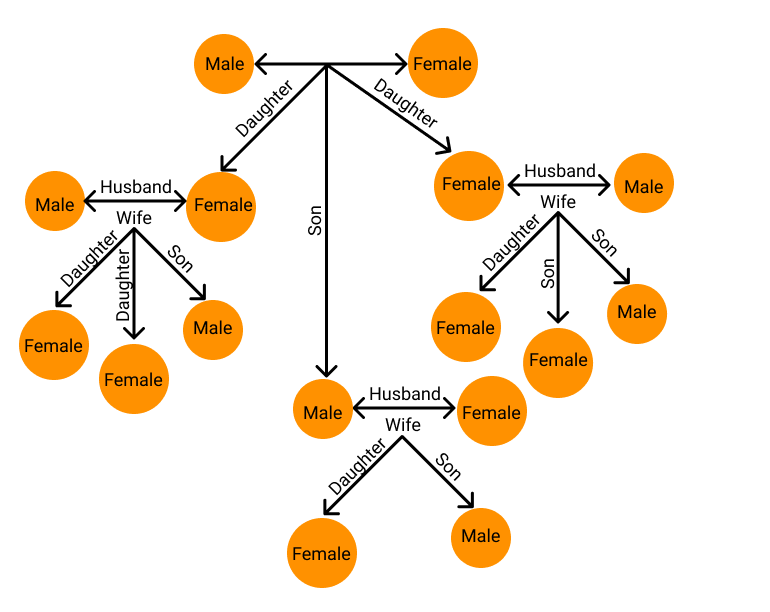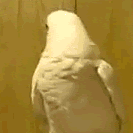# Introduction to GraphsNeelakshi Lahiri
Last Updated: May 13, 2022
Difficulty Level :
EASY

## Introduction

When we hear the word graph, what comes to our mind?

Something like this?Graphs in data structures aren’t like that, though. Then what are they?To understand graphs in data structures, let us consider a family tree.

In a family tree, we can see different family members and the relations between them.Graphs in data structures are similar where each member of the family is called a node, and their relationships are called edges.

Thus, to formally define it,

A graph is a non-linear data structure containing points called nodes and links between them called edges.

In this article, we’ll learn the basics terminologies of graph data structure and its types.

## Basic Terminologies

To begin with, let’s see some basic terms related to graphs in data structures.

Let’s transform the family tree above into a generalised graph.### Vertex or Node

Each point or element in a graph is known as a vertex or node.

In our example above, each family member is a vertex or node.

### Edge or Arc

The link connecting two nodes is called an edge or an arc.

In the graph above, the relations between the members are the edges or arcs.

### End vertices or endpoints

The two nodes joined by an edge are called the end vertices or endpoints of that edge. For example, for the edge between the grandparents, the endpoints are grandma and grandpa.

### Origin

Notice in the family tree above that the link between our parents and us is shown with a unidirectional array. Such an edge is called a directed edge.

In such edges, the first endpoint (here our parents) is known as the origin.

### Destination

In the same case of a directed edge as discussed for an origin, the node containing our siblings and us is called the directed edge’s destination.

In the example above, if there is a node for a neighbour of ours, then will they be related to us?Source: giphy

Thus, there won’t be any edge between any of our family members or them, but there is an edge between everyone in the same family (although all the edges have not been shown to keep the diagram simple). So, two nodes with an edge are said to be adjacent, and the nodes are called neighbours.

### Incident

In our graph above, all the edges are pointing to a node. So, a node is the endpoint of every edge. In technical terms, the edge is said to be incident on a vertex.

### Outgoing Edge

We saw what the origin is. So there, the edge is pointing away from the origin and is called an outgoing edge.

### Incoming Edge

From the destination end, the edge is called an incoming edge.

### Parallel Edges or Multiple Edges

In graph theory, parallel edges (also called multiple edges or a multi-edge), are two or more edges that are incident to the same two vertices.

### Self Loop

If a node has an edge such that it is both the source and destination, it is called a self-loop. To understand this better, let us see a picture.

### Degree

The degree is the total number of edges connected to a node.

For example, in the example of a family tree, let us consider just ourselves. We are our parents’ kids, our aunt and uncle’s niece or nephew, our grandparents’ grandchild,  and our siblings and cousins’ sister or brother.

.

So here, the degree of the node “You” is 11

### In-degree

To understand this, let us consider our previous example again. There, all the edges are directed towards the “you” node. So, the total number of incoming edges for that node is 11. This number is called the in-degree.

### Out-degree

Considering the previous example again, if we consider the opposite relation, i.e., our family includes grandparents, parents, an aunt, an uncle and cousins. The graph for this would be as follows:

Here, all the edges are outgoing edges, and there are 11 of them. So the out-degree of the “you” node is 11.

### Path

Almost all of us have been to a family occasion where we meet a relative and fail to recognise them.

We then try to figure it out by relating who is related to whom. Suppose we want to figure out we are related to a particular cousin, then we will follow the path shown below:

Mom ➡ Grandma and Grandpa ➡ Uncle ➡ Sister

Thus, we can define a path as alternate nodes and edges, leading us to another node.

### Bridge

If our grandparents didn’t exist in our family tree example, would our aunt, uncle and mom or any subsequent generations exist? Of course not. Similarly, in a graph, the node, which on removing makes the graph disconnected, is called the bridge.

### Tree

Let us first see a picture as follows:

.

A graph like this is known as a cycle graph.

A graph without any such cycle is called a tree. For example,

### Forest

A connected graph with many trees is called a forest.

Now that we know some basics of graph data structures, let us learn about the different types of graphs.

## Types of Graphs

Graphs can be of different types. In all, there are 15 types of graphs. Let’s see what they are.

### Null Graph

Consider a random group of people who are meeting for the first time. They do not know each other, and so there is no relation between them.

If such data is represented in a graph, it will have only independent nodes which are not linked by any edges. Such graphs in data structures are called null graphs.

### Trivial Graph

Consider a single person.

If that person is a node, then a graph with a single node is called a trivial graph. This is the simplest graph possible.

### Connected Graph

Now let us consider that the people in the group soon became friends. So now, there will be edges between them.

Here, notice that all the nodes are accessible through some path.

Thus a graph in which all the nodes are connected such that they are accessible through some path is called a connected graph.

### Disconnected Graph

Consider the same example again. If one (or even more than one) of the friends leave the group, we remove the corresponding edge(s). Suppose Kendra left the group.

Now the node “Kendra” is not connected to the rest of the nodes, and we cannot reach it from any of the nodes. Such a graph is called a disconnected graph.

Thus, a graph in which one or more nodes are not connected so that any path cannot reach them is called a disconnected graph.

### Unweighted Graph

In all the graphs we saw above, the edges of the graphs did not have a value of their own. In other words, the edges did not have weights. Such graphs are called unweighted graphs.

### Weighted Graph

We just learned that if the edges of a graph don’t have weights, they are called unweighted graphs. Then what if they do have weights as shown below?

Graphs in which the edges have weights are known as weighted graphs. Practically, they are used to map the distance between two locations.

### Undirected Graph

In the graph shown above, did you notice that the edges were not directed? I’m sure you did, and such a graph has a particular name.

A graph in which the edges are not directed is called an undirected graph.

### Directed Graph

Now, let us assume that these people are colleagues in an office. Some of them are managers, and the others are employees under them. So, the employees will have to report to their respective managers. To show that, we’ll use directed edges. Such a graph is known as a directed graph.

### Simple Graph

A graph in which all the edges connect two different nodes is called a simple graph.

All the graphs we have seen so far are examples of simple graphs.

### Multigraph

A graph containing self-loops is called a multigraph. For example,

### Complete Graph

Considering the colleagues in the office again, let us assume that four people, in particular, have become great friends amongst themselves. So naturally, they all know each other.

Such a graph in which each node is connected to every other node is called a complete graph.

### Regular Graph

Remember learning about the degree of a graph before? Let’s use that knowledge now to learn what a regular graph is.

A graph in which the degree of all the nodes is the same is called a regular graph.

An example of a regular graph is shown below:

### Cyclic Graph

A cyclic graph is one that has at least one graph cycle. A unicyclic graph is a cyclic graph with exactly one (undirected, simple) cycle.

While learning about trees and forests, we read that a small group of nodes without a cycle is a tree, while connected trees are called forests. Hence, trees are not cyclic graphs.

### Directed Acyclic Graph

To understand this, let me first show you the picture of a graph.

At first glance, doesn’t it look like a cycle graph?

Source: giphy

If you notice carefully, it isn’t a cycle graph. It is a directed acyclic graph, which has directed edges but no cycles.

### Bipartite Graph

Among the group of people mentioned above, suppose we divide them into managers and workers. Now, the managers will have workers under them. Neither the workers nor the managers will report to each other. This arrangement can be represented by a graph as follows:

A graph like this is called a bipartite graph. Thus, to formally define it,

A Bipartite Graph is one in which the vertices can be divided into two independent sets, U and V, and each edge (u, v) connects either a vertex from U to V or a vertex from V to U.

## FAQs

1. What is a graph?
A graph is a non-linear data structure containing points called nodes and links between them called edges.

2. Why are graphs important data structures?
The graphs in data structures are important since they can easily represent the relations between real-life data.

3. What is a practical application of graph data structure?
In navigation, graphs are practically used to map the shortest distance between two cities by a computer.

4. What is the difference between a tree and a graph?
tree is a special kind of graph that contains no cycles. So, every tree is a graph, but all graphs are not trees.

5. What are the types of graphs in data structures?
The types of graphs are:
• Null graph
• Trivial graph
• Connected and Disconnected graph
• Directed and Undirected graph
• Simple graph
• Multigraph
• Complete graph
• Regular graph
• Cyclic graph
• Directed Acyclic graph
• Weighted and Unweighted graph
• Bipartite graph

## Key Takeaways

In this article, we learned about just the basics of graphs in data structures and algorithms, but this is just the beginning.

With the help of these basics, we can now theoretically understand the concept of graphs better. Our next job will be to learn how graphs are represented and practically implement them.

So, if you’re confident about all the terminologies of graphs in data structures, let’s move on to the next article on Graph representation .

At last, we summarised the entire article as well as additional topics in a short video for your convenience in learning graphs.